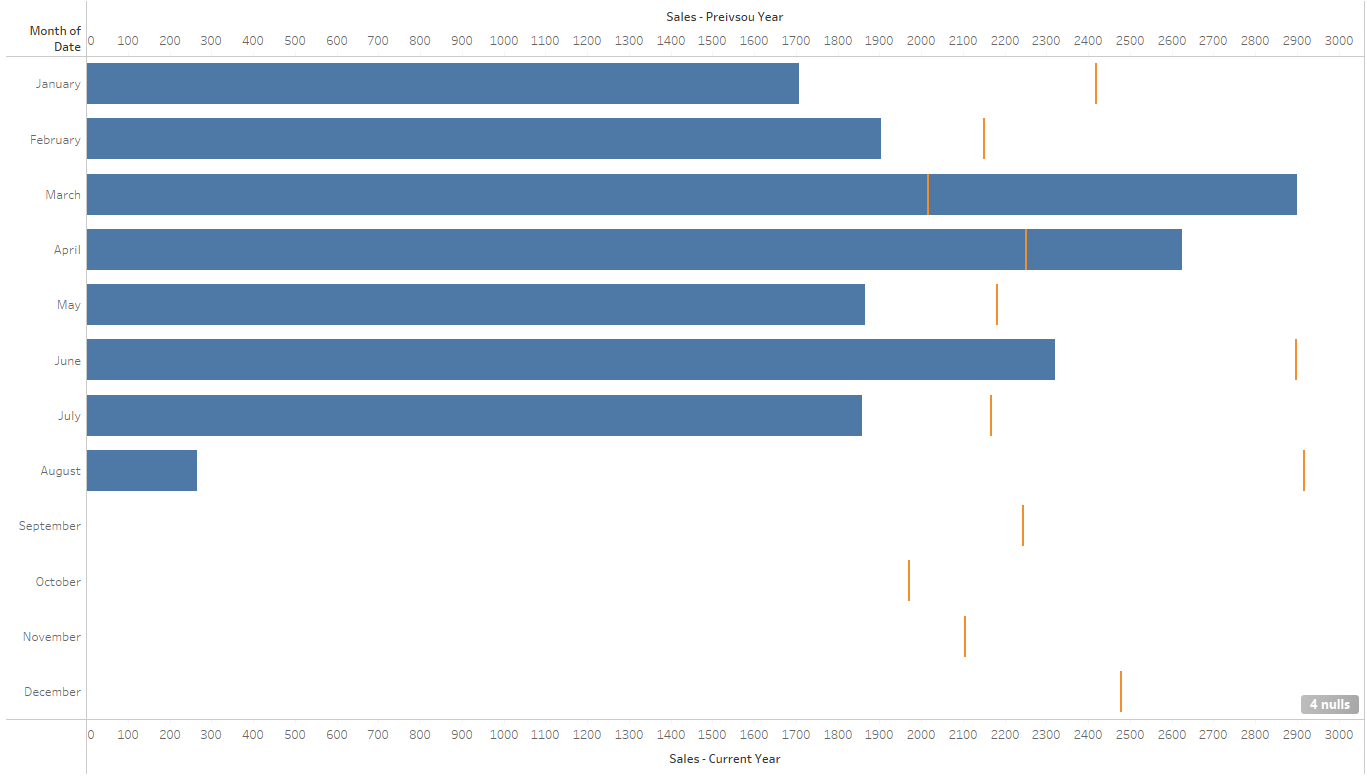## Year on Year Comparison Part 1 - Gantt Chart in Tableau

by Liu Zhang

Recently I have encountered an issue to present the year on year (YoY) trend comparison, although there are ways to show the trend, none of them are the most satisfactory.

### A quick way:

It is easy to create a line chart, then split by year, but it is not easy to compare two separate line chart together and the the axis are different range if the date is incomplete for one period.

### A better way:A Gantt chart that uses the previous year as reference bar

A better way to compare YoY trend is to use a Gantt Chart, where the current year values are shown as bars (if exist) and the previous year values are shown as reference bar.

Note: Here the view is broken down by (discrete) Month, in practice, the view can be broken down by any discrete field.

To make the calculation more dynamic that doesn't depend on the future manual adjustment, we will try to use date calculation functions within Tableau rather than manually set the dates.

Note: The blog will aggregate the calculation to monthly level, though any other level can be used depends on the requirement or we can even setup a parameter to control the level (blog link).

First we will create the benchmark date, which is the latest date available for a given dataset, such as sales date, invoice date, delivery date etc.

Then we can find the first day of the year for the given End Date calculated, by using DATETRUNC() function.

The latest date within the sample dataset is 5th Aug 2021, then the calculation will truncate the date to (year) 2021, as the function will return a field that is still a Date type field, the exact output will be 1st Jan 2021.

Next we can find the previous year by subtract 1 year from the current latest date, then truncate the date. To subtract date in Tableau, we use DATEADD() function.

By above logic, the calculation will return 1st Jan 2020.

With the reference dates calculated, we can start define Current Year (CY) and Previous Year (PY) by using those dates.

Since we are (usually) interested in the latest YoY comparison, we will drag the Date - Year Indicator to Filter and filter out More than Previous Year.

Next we will use similar calculation to calculate the measure values, (Sales in this example) to be used in the view.

To check the above calculations are correct, we can display the result in the table format.

Once we can confirm the calcualtion is correct, we can start to setup the view by dragging the label into the corresponding place.

This should present the following view, where people are free to format the colour, size and tooltips themself.

Looking for more guides, tips and tricks in Tableau or Alteryx? Go check out the other blog posts from the Data School.

Tableau Public